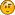###Poll

#### how can i calculate the following line?1 (100%)0 (0%)

Total Members Voted: 1

###Author Topic: Mathematical Problem  (Read 2688 times)

#### si20596

• Newbie
•• Posts: 4##### Mathematical Problem
« on: July 30, 2013, 01:32:09 am »
Hello all,

how can i calculate the following line?

tmp = 1234567.12345 + 34567.45678 * 12 + 0.00123 * 12 * 12 + 0.00333 * 12 ^ 3 - 0.0022

#### stanl##### Re: Mathematical Problem
« Reply #1 on: July 30, 2013, 06:50:29 am »
Not sure about the grouping, but you can practice by putting the formula into Excel. See attached#### td##### Re: Mathematical Problem
« Reply #2 on: July 30, 2013, 07:31:11 am »
First, you need to use the WinBatch Exponentiation operator

Code: Winbatch
tmp = 1234567.12345 + 34567.45678 * 12 + 0.00123 * 12 * 12 + 0.00333 * 12 ** 3 - 0.0022

and as Stan mentioned you may need to add parenthesizes to change the operator evaluation order.  It all depends on the purpose of  the expression.
"No one who sees a peregrine falcon fly can ever forget the beauty and thrill of that flight."